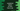# JavaScript program to delete an item from a set## How to delete an item from a set in JavaScript:

In this post, we will learn how to delete an item from a set in JavaScript with different examples. Set object provides a method to delete items. It is called delete. In this post, we will learn the definition of this method and how to use it with examples.

### Definition of delete:

delete() method is defined as like below:

``delete(v)``

Here, v is the value to remove from the Set. It returns one boolean value. If it is true, the value was in the set and it was removed. If it is false, the value was not in the set.

## Example of delete:

Let’s take an example of delete on how it works:

``````let givenSet = new Set();

console.log('Given set: ', givenSet);

console.log('Return value of delete: ',givenSet.delete('World'));

console.log('Final set: ',givenSet);``````

Here,

• givenSet is an empty set and we are using add to add two words to it.
• The first log statement is printing the set before any item is deleted. The second log statement is printing the return value for delete and the third log statement is printing the set again after the item is deleted.

If you run this program, it will print the below output:

``````Given set:  Set(2) { 'Hello', 'World' }
Return value of delete:  true
Final set:  Set(1) { 'Hello' }``````

## Example with multiple values:

Let’s try this with multiple values to delete from a set. We will create one program that will keep deleting numbers from a given set. It will print one message if the deletion is successful or not.

Below is the complete program:

``````let givenSet = new Set();

for(let i = 1; i<= 10; i++){
}

let testSet = [1, 3, 22, 6, 44, 1]

console.log('Given set: ',givenSet);

for(let item of testSet){
if(givenSet.delete(item)){
console.log(`\${item} is deleted`);
}
else{
console.log(`\${item} is not found in the set`);
}
}

console.log('Final set: ',givenSet);``````
• givenSet is a set that holds all numbers from 1 to 10.
• testSet is a list of numbers we are deleting from givenSet.
• The for loop iterates through the items of testSet one by one and it uses delete to delete the items from givenSet. Based on the return value of delete, it prints one message, i.e. the item is deleted or not.
• After the for loop is done, it is printing the same set givenSet again.

If you run this program, it will print the below output:

``````Given set:  Set(10) { 1, 2, 3, 4, 5, 6, 7, 8, 9, 10 }
1 is deleted
3 is deleted
22 is not found in the set
6 is deleted
44 is not found in the set
1 is not found in the set
Final set:  Set(7) { 2, 4, 5, 7, 8, 9, 10 }``````

As you can see here, 1, 3, and 6 are deleted from the set. 1 is two times in the list. But, it deletes only for one time, i.e. delete returns true for the first occurrence and returns false for the second occurrence.

## Example with objects:

Let’s try another example with different objects. Consider the below example:

``````let givenSet = new Set();

givenSet.add({name: 'Alex', age: 20});

console.log(givenSet.delete({name: 'Alex', age: 20}));``````

We have added one object to the set givenSet and we are trying to delete an object with the same name and age as the object added. But, it will return false because both are not referring to the same object.

But, if we use the reference to the same object, it will work.

``````let givenSet = new Set();

let student = {name: 'Alex', age: 20};

console.log(givenSet.delete(student));``````

It will print true.

For a set with multiple values, we can get the reference if we use a loop. For example:

``````let givenSet = new Set();

givenSet.add({name: 'Alex', age: 20});
givenSet.add({name: 'Bob', age: 14});
givenSet.add({name: 'Chandler', age: 10});
givenSet.add({name: 'Emily', age: 23});

console.log('Given set: ',givenSet);

for(let item of givenSet){
if(item.age < 18){
givenSet.delete(item);
}
}

console.log('Final set: ',givenSet);``````

In this example, we are adding four different objects to givenSet and by using a for loop, we are removing all objects which have age smaller than 18.

If you run this program, it will print the below output:

``````Given set:  Set(4) {
{ name: 'Alex', age: 20 },
{ name: 'Bob', age: 14 },
{ name: 'Chandler', age: 10 },
{ name: 'Emily', age: 23 }
}
Final set:  Set(2) { { name: 'Alex', age: 20 }, { name: 'Emily', age: 23 } }``````